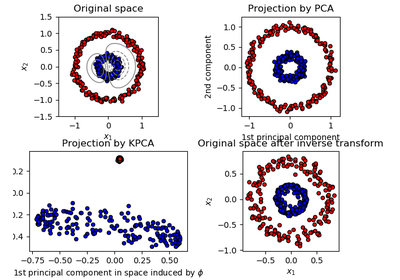# `sklearn.decomposition`.KernelPCA¶

class `sklearn.decomposition.``KernelPCA`(n_components=None, kernel=’linear’, gamma=None, degree=3, coef0=1, kernel_params=None, alpha=1.0, fit_inverse_transform=False, eigen_solver=’auto’, tol=0, max_iter=None, remove_zero_eig=False, random_state=None, copy_X=True, n_jobs=1)[source]

Kernel Principal component analysis (KPCA)

Non-linear dimensionality reduction through the use of kernels (see Pairwise metrics, Affinities and Kernels).

Read more in the User Guide.

Parameters: n_components : int, default=None Number of components. If None, all non-zero components are kept. kernel : “linear” | “poly” | “rbf” | “sigmoid” | “cosine” | “precomputed” Kernel. Default=”linear”. gamma : float, default=1/n_features Kernel coefficient for rbf, poly and sigmoid kernels. Ignored by other kernels. degree : int, default=3 Degree for poly kernels. Ignored by other kernels. coef0 : float, default=1 Independent term in poly and sigmoid kernels. Ignored by other kernels. kernel_params : mapping of string to any, default=None Parameters (keyword arguments) and values for kernel passed as callable object. Ignored by other kernels. alpha : int, default=1.0 Hyperparameter of the ridge regression that learns the inverse transform (when fit_inverse_transform=True). fit_inverse_transform : bool, default=False Learn the inverse transform for non-precomputed kernels. (i.e. learn to find the pre-image of a point) eigen_solver : string [‘auto’|’dense’|’arpack’], default=’auto’ Select eigensolver to use. If n_components is much less than the number of training samples, arpack may be more efficient than the dense eigensolver. tol : float, default=0 Convergence tolerance for arpack. If 0, optimal value will be chosen by arpack. max_iter : int, default=None Maximum number of iterations for arpack. If None, optimal value will be chosen by arpack. remove_zero_eig : boolean, default=False If True, then all components with zero eigenvalues are removed, so that the number of components in the output may be < n_components (and sometimes even zero due to numerical instability). When n_components is None, this parameter is ignored and components with zero eigenvalues are removed regardless. random_state : int, RandomState instance or None, optional (default=None) If int, random_state is the seed used by the random number generator; If RandomState instance, random_state is the random number generator; If None, the random number generator is the RandomState instance used by np.random. Used when `eigen_solver` == ‘arpack’. New in version 0.18. copy_X : boolean, default=True If True, input X is copied and stored by the model in the X_fit_ attribute. If no further changes will be done to X, setting copy_X=False saves memory by storing a reference. New in version 0.18. n_jobs : int, default=1 The number of parallel jobs to run. If -1, then the number of jobs is set to the number of CPU cores. New in version 0.18. lambdas_ : array, (n_components,) Eigenvalues of the centered kernel matrix in decreasing order. If n_components and remove_zero_eig are not set, then all values are stored. alphas_ : array, (n_samples, n_components) Eigenvectors of the centered kernel matrix. If n_components and remove_zero_eig are not set, then all components are stored. dual_coef_ : array, (n_samples, n_features) Inverse transform matrix. Set if fit_inverse_transform is True. X_transformed_fit_ : array, (n_samples, n_components) Projection of the fitted data on the kernel principal components. X_fit_ : (n_samples, n_features) The data used to fit the model. If copy_X=False, then X_fit_ is a reference. This attribute is used for the calls to transform.

References

Kernel PCA was introduced in:
Bernhard Schoelkopf, Alexander J. Smola, and Klaus-Robert Mueller. 1999. Kernel principal component analysis. In Advances in kernel methods, MIT Press, Cambridge, MA, USA 327-352.

Methods

 `fit`(X[, y]) Fit the model from data in X. `fit_transform`(X[, y]) Fit the model from data in X and transform X. `get_params`([deep]) Get parameters for this estimator. `inverse_transform`(X) Transform X back to original space. `set_params`(**params) Set the parameters of this estimator. `transform`(X) Transform X.
`__init__`(n_components=None, kernel=’linear’, gamma=None, degree=3, coef0=1, kernel_params=None, alpha=1.0, fit_inverse_transform=False, eigen_solver=’auto’, tol=0, max_iter=None, remove_zero_eig=False, random_state=None, copy_X=True, n_jobs=1)[source]
`fit`(X, y=None)[source]

Fit the model from data in X.

Parameters: X : array-like, shape (n_samples, n_features) Training vector, where n_samples in the number of samples and n_features is the number of features. self : object Returns the instance itself.
`fit_transform`(X, y=None, **params)[source]

Fit the model from data in X and transform X.

Parameters: X : array-like, shape (n_samples, n_features) Training vector, where n_samples in the number of samples and n_features is the number of features. X_new : array-like, shape (n_samples, n_components)
`get_params`(deep=True)[source]

Get parameters for this estimator.

Parameters: deep : boolean, optional If True, will return the parameters for this estimator and contained subobjects that are estimators. params : mapping of string to any Parameter names mapped to their values.
`inverse_transform`(X)[source]

Transform X back to original space.

Parameters: X : array-like, shape (n_samples, n_components) X_new : array-like, shape (n_samples, n_features)

References

“Learning to Find Pre-Images”, G BakIr et al, 2004.

`set_params`(**params)[source]

Set the parameters of this estimator.

The method works on simple estimators as well as on nested objects (such as pipelines). The latter have parameters of the form `<component>__<parameter>` so that it’s possible to update each component of a nested object.

Returns: self :
`transform`(X)[source]

Transform X.

Parameters: X : array-like, shape (n_samples, n_features) X_new : array-like, shape (n_samples, n_components)

## Examples using `sklearn.decomposition.KernelPCA`¶Kernel PCA